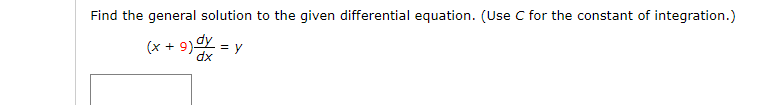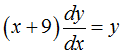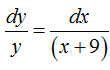# Find the general solution to the given differential equation. (Use C for the constant of integration.)(x + 9)Y = ydx

Question
1 viewshelp_outlineImage TranscriptioncloseFind the general solution to the given differential equation. (Use C for the constant of integration.) (x + 9)Y = y dx fullscreen
check_circle

star
star
star
star
star
1 Rating
Step 1

Consider the given differential equation.Step 2

Rewrite the above differential equation as,...

### Want to see the full answer?

See Solution

#### Want to see this answer and more?

Solutions are written by subject experts who are available 24/7. Questions are typically answered within 1 hour.*

See Solution
*Response times may vary by subject and question.
Tagged in